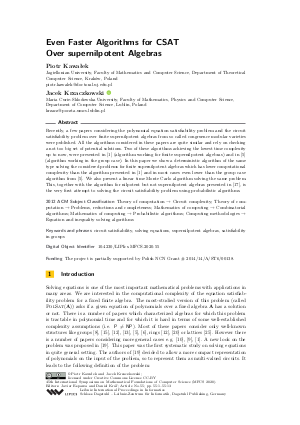Document# Even Faster Algorithms for CSAT Over supernilpotent Algebras

### Authors Piotr Kawałek, Jacek Krzaczkowski## File

LIPIcs.MFCS.2020.55.pdf
• Filesize: 483 kB
• 13 pages

## Cite As

Piotr Kawałek and Jacek Krzaczkowski. Even Faster Algorithms for CSAT Over supernilpotent Algebras. In 45th International Symposium on Mathematical Foundations of Computer Science (MFCS 2020). Leibniz International Proceedings in Informatics (LIPIcs), Volume 170, pp. 55:1-55:13, Schloss Dagstuhl - Leibniz-Zentrum für Informatik (2020)
https://doi.org/10.4230/LIPIcs.MFCS.2020.55

## Abstract

Recently, a few papers considering the polynomial equation satisfiability problem and the circuit satisfiability problem over finite supernilpotent algebras from so called congruence modular varieties were published. All the algorithms considered in these papers are quite similar and rely on checking a not too big set of potential solutions. Two of these algorithms achieving the lowest time complexity up to now, were presented in [Aichinger, 2019] (algorithm working for finite supernilpotent algebras) and in [Földvári, 2018] (algorithm working in the group case). In this paper we show a deterministic algorithm of the same type solving the considered problems for finite supernilpotent algebras which has lower computational complexity than the algorithm presented in [Aichinger, 2019] and in most cases even lower than the group case algorithm from [Földvári, 2018]. We also present a linear time Monte Carlo algorithm solving the same problem. This, together with the algorithm for nilpotent but not supernilpotent algebras presented in [Paweł M. Idziak et al., 2020], is the very first attempt to solving the circuit satisfiability problem using probabilistic algorithms.

## Subject Classification

##### ACM Subject Classification
• Theory of computation → Circuit complexity
• Theory of computation → Problems, reductions and completeness
• Mathematics of computing → Combinatorial algorithms
• Mathematics of computing → Probabilistic algorithms
• Computing methodologies → Equation and inequality solving algorithms
##### Keywords
• circuit satisfiability
• solving equations
• supernilpotent algebras
• satisfiability in groups

## Metrics

• Access Statistics
• Total Accesses (updated on a weekly basis)
0

## References

1. Erhard Aichinger. Solving systems of equations in supernilpotent algebras. arXiv preprint arXiv:1901.07862, 2019.2. Erhard Aichinger and Nebojša Mudrinski. Some applications of higher commutators in Mal'cev algebras. Algebra universalis, 63(4):367-403, 2010.3. Andrei Bulatov. On the number of finite Mal’tsev algebras. Contributions to general algebra, 13:41-54, 2000.4. Stanley Burris and Hanamantagouda P. Sankappanavar. A Course in Universal Algebra. Dover Publications, 2014.5. Attila Földvári. The complexity of the equation solvability problem over nilpotent groups. Journal of Algebra, 495:289–303, 2018.6. Attila Földvári and Gábor Horváth. The complexity of the equation solvability and equivalence problems over finite groups. International Journal of Algebra and Computation, 30(3):1-17, 2019.7. Ralph Freese and Ralph McKenzie. Commutator theory for congruence modular varieties, volume 125. CUP Archive, 1987.8. Mikael Goldmann and Alexander Russell. The complexity of solving equations over finite groups. Information and Computation, 178(1):253-262, 2002.9. Tomasz Gorazd and Jacek Krzaczkowski. Term equation satisfiability over finite algebras. International Journal of Algebra and Computation, 20(8):1001-1020, 2010.10. Tomasz Gorazd and Jacek Krzaczkowski. The complexity of problems connected with two-element algebras. Reports on Mathematical Logic, 46:91-108, 2011.11. Johan Håstad. Satisfying degree-d equations over GF. Theory of Computing, 9:845–862, 2013.12. Gábor Horváth. The complexity of the equivalence and equation solvability problems over nilpotent rings and groups. Algebra universalis, 66(4):391-403, 2011.13. Gábor Horváth. The complexity of the equivalence and equation solvability problems over meta-abelian groups. Journal of Algebra, 433:208-230, 2015.14. Gábor Horváth and Csaba Szabó. The extended equivalence and equation solvability problems for groups. Discrete Mathematics and Theoretical Computer Science, 13(4):23-32, 2011.15. Gábor Horváth and Csaba Szabó. The complexity of checking identities over finite groups. International Journal of Algebra and Computation, 16(5):931-940, 2006.16. Paweł M. Idziak, Piotr Kawałek, and Jacek Krzaczkowski. Expressive power, satisfiability and equivalence of circuits over nilpotent algebras. In 43rd International Symposium on Mathematical Foundations of Computer Science (MFCS 2018). Schloss Dagstuhl-Leibniz-Zentrum fuer Informatik, 2018.17. Paweł M. Idziak, Piotr Kawałek, and Jacek Krzaczkowski. Intermediate problems in modular circuits satisfiability. In Proceedings of the 35th Annual ACM/IEEE Symposium on Logic in Computer Science, 2020.18. Paweł M. Idziak, Piotr Kawałek, and Jacek Krzaczkowski. Stratifying algebras by supernilpotent intervals. Manuscript, 2020.19. Paweł M. Idziak and Jacek Krzaczkowski. Satisfiability in multi-valued circuits. In Proceedings of the 33rd Annual ACM/IEEE Symposium on Logic in Computer Science, pages 550-558. ACM, 2018.20. Gyula Károlyi and Csaba Szabó. The complexity of the equation solvablity problem over nilpotent rings. Manuscript available at http://web.cs.elte.hu/asciitilde csaba/publications, 2015.21. Piotr Kawałek, Michael Kompatscher, and Jacek Krzaczkowski. Circuit equivalence in 2-nilpotent algebras. arXiv preprint arXiv:1909.12256, 2019.22. Keith Kearnes. Congruence modular varieties with small free spectra. Algebra Universalis, 42(3):165-181, 1999.23. Michael Kompatscher. The equation solvability problem over supernilpotent algebras with Mal’cev term. International Journal of Algebra and Computation, 28(6):1005-1015, 2018.24. Michael Kompatscher. CC-circuits and the expressive power of nilpotent algebras. arXiv preprint arXiv:1911.01479, 2019.25. Bernhard Schwarz. The complexity of satisfiability problems over finite lattices. In Annual Symposium on Theoretical Aspects of Computer Science, pages 31-43, 2004.26. Joel VanderWerf. Wreath decomposition of algebras. PhD thesis, University of California, Berkeley, 1995.X

Feedback for Dagstuhl Publishing